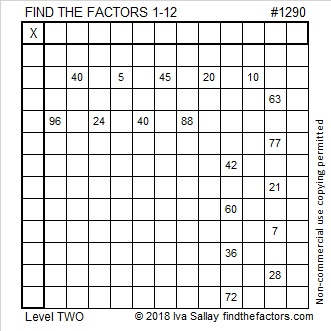# 1290 Multiplication Boomerang

Do multiplication and division facts seem like something you threw out long ago but still come back to hit you? Perhaps this puzzle can help you get more familiar with those facts so they won’t hurt you so much anymore.Print the puzzles or type the solution in this excel file: 12 factors 1289-1299

That was puzzle number 1290. Here are some facts about that number:

• 1290 is a composite number.
• Prime factorization: 1290 = 2 × 3 × 5 × 43
• The exponents in the prime factorization are 1, 1, 1, and 1. Adding one to each and multiplying we get (1 + 1)(1 + 1)(1 + 1)(1 + 1) = 2 × 2 × 2 × 2 = 16. Therefore 1290 has exactly 16 factors.
• Factors of 1290: 1, 2, 3, 5, 6, 10, 15, 30, 43, 86, 129, 215, 258, 430, 645, 1290
• Factor pairs: 1290 = 1 × 1290, 2 × 645, 3 × 430, 5 × 258, 6 × 215, 10 × 129, 15 × 86, or 30 × 43
• 1290 has no square factors that allow its square root to be simplified. √1290 ≈ 35.916571290 is the sum of two consecutive prime numbers:
643 + 647 = 1290

1290 is the hypotenuse of a Pythagorean triple:
774-1032-1290 which is (3-4-5) times 258

This site uses Akismet to reduce spam. Learn how your comment data is processed.